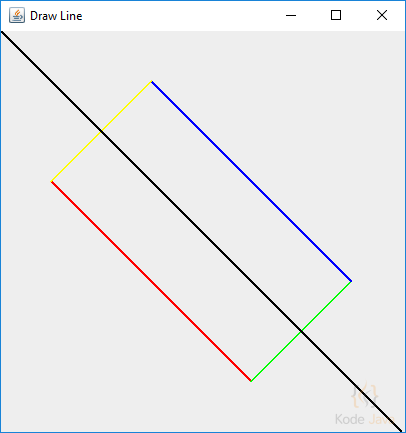# How do I draw a line in Java 2D?

The following code snippet show you how to draw a simple line using `Graphics2D.draw()` method. This method take a parameter that implements the `java.awt.Shape` interface.

To draw a line we can use the `Line2D.Double` static-inner class. This class constructor takes four integers values that represent the start `(x1, y1)` and end `(x2, y2)` coordinate of the line.

``````package org.kodejava.example.geom;

import javax.swing.*;
import java.awt.*;
import java.awt.geom.Line2D;

public class DrawLine extends JComponent {
@Override
public void paint(Graphics g) {
// Draw a simple line using the Graphics2D draw() method.
Graphics2D g2 = (Graphics2D) g;
g2.setStroke(new BasicStroke(2f));
g2.setColor(Color.RED);
g2.draw(new Line2D.Double(50, 150, 250, 350));
g2.setColor(Color.GREEN);
g2.draw(new Line2D.Double(250, 350, 350, 250));
g2.setColor(Color.BLUE);
g2.draw(new Line2D.Double(350, 250, 150, 50));
g2.setColor(Color.YELLOW);
g2.draw(new Line2D.Double(150, 50, 50, 150));
g2.setColor(Color.BLACK);
g2.draw(new Line2D.Double(0, 0, 400, 400));
}

public static void main(String[] args) {
JFrame frame = new JFrame("Draw Line");
frame.setDefaultCloseOperation(JFrame.EXIT_ON_CLOSE);
frame.pack();
frame.setSize(new Dimension(420, 440));
frame.setVisible(true);
}
}
``````

When you run the snippet it will show you something like:1.Phanxico 13
•Wayan Saryada
If you want to change the thickness of the line you can change the value of the stroke size that is used to draw the line. See the `g2.setStroke()` line in the code snippet above.